Miscellaneous

Chapter 9 Class 11 Sequences and Series
Serial order wise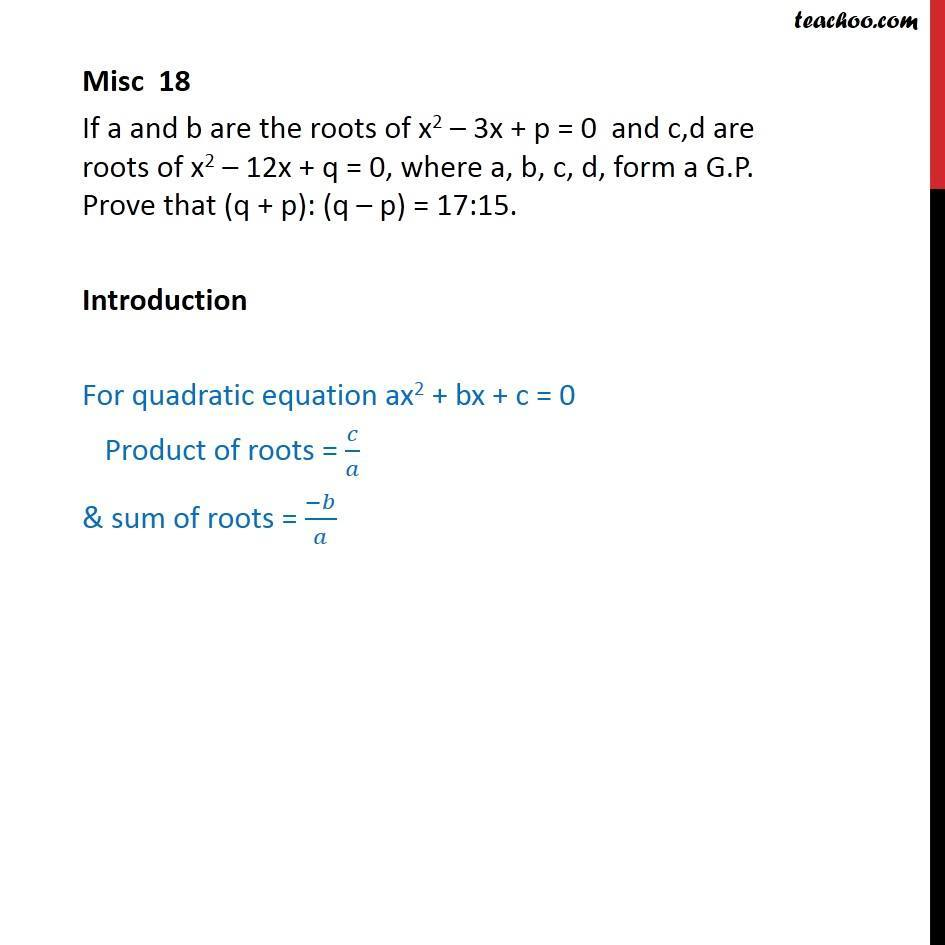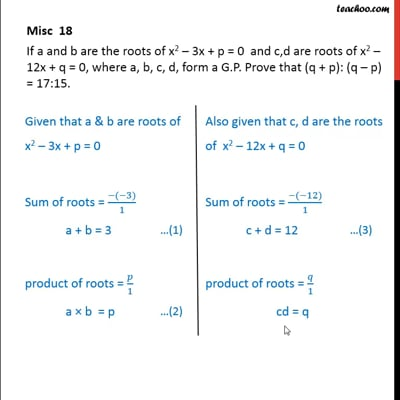This video is only available for Teachoo black users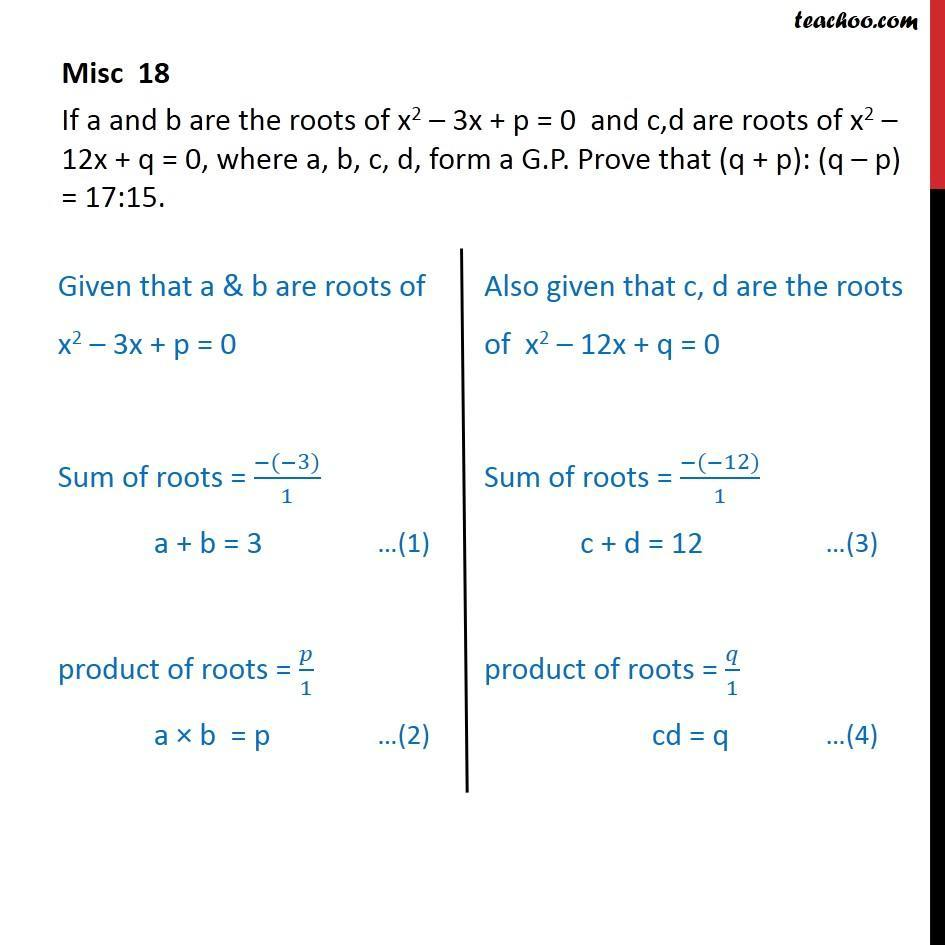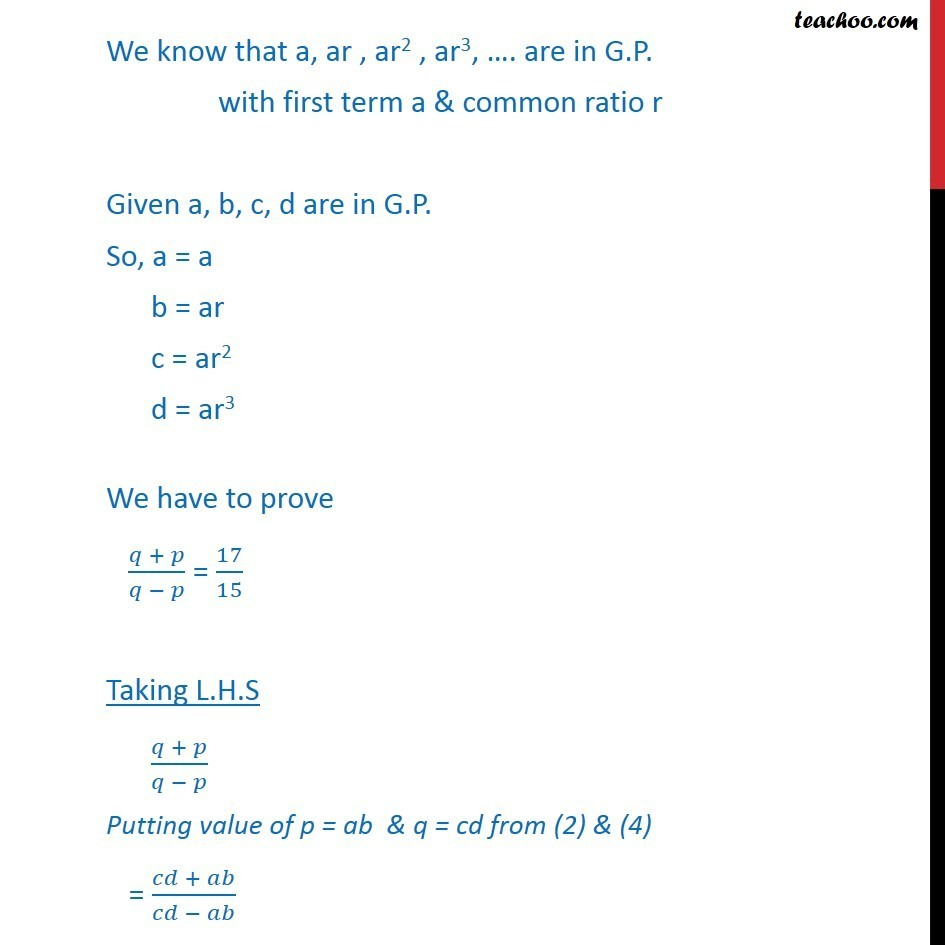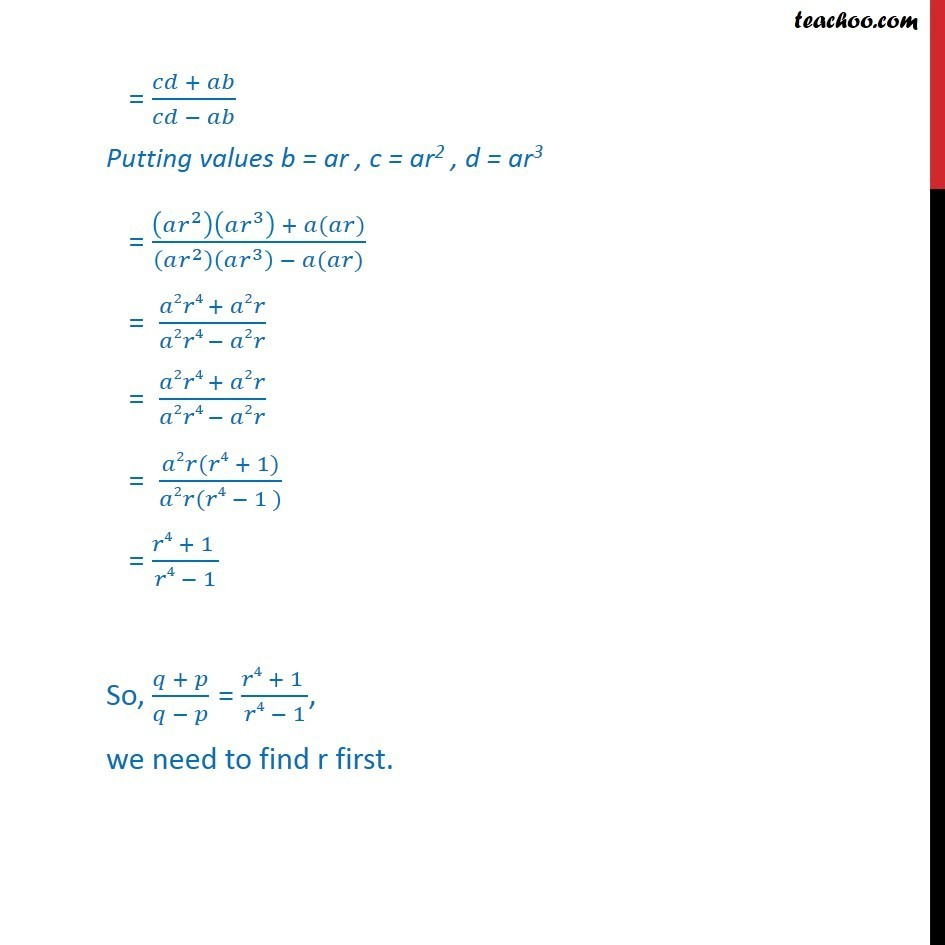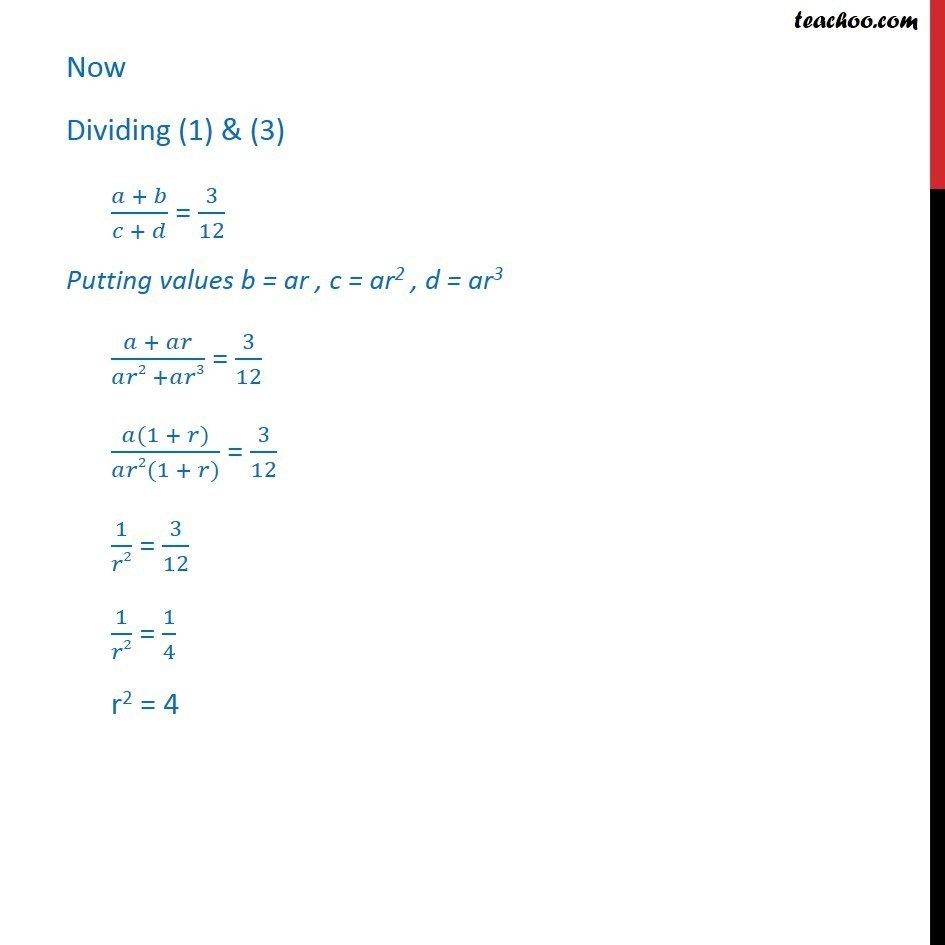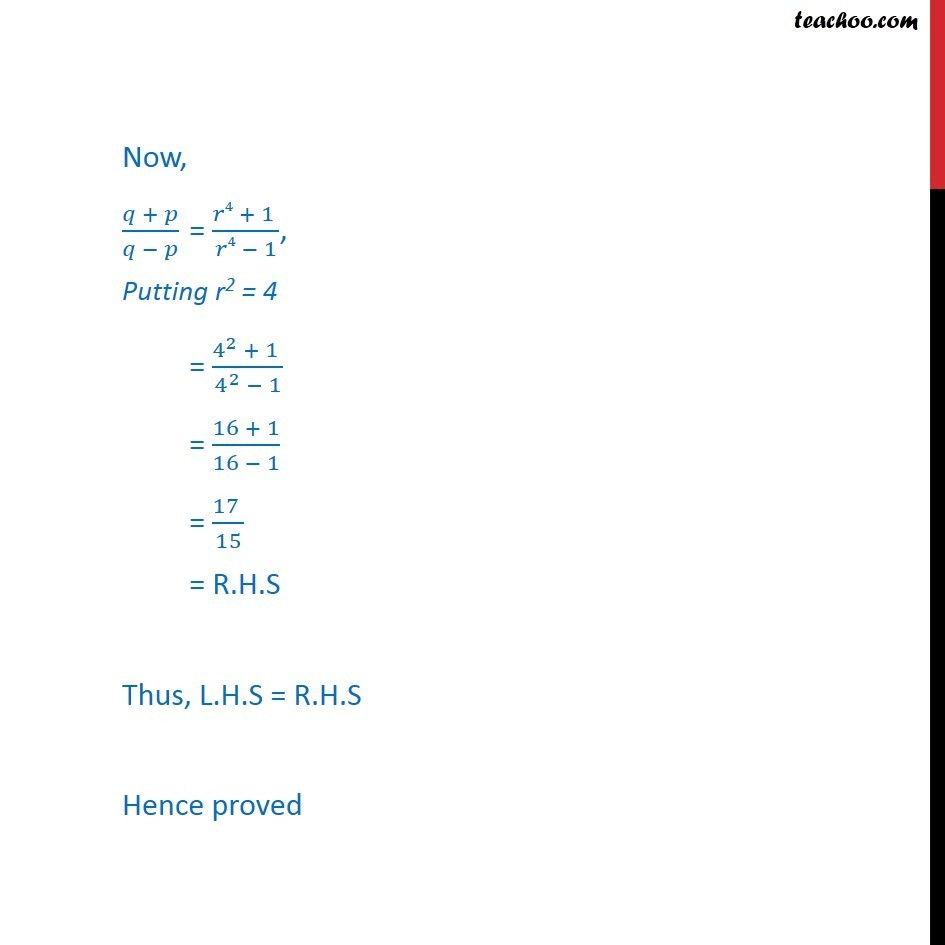This video is only available for Teachoo black users

Solve all your doubts with Teachoo Black (new monthly pack available now!)

### Transcript

Misc 18 If a and b are the roots of x2 – 3x + p = 0 and c,d are roots of x2 – 12x + q = 0, where a, b, c, d, form a G.P. Prove that (q + p): (q – p) = 17:15. Introduction For quadratic equation ax2 + bx + c = 0 Product of roots = 𝑐/𝑎 & sum of roots = (−𝑏)/𝑎 Misc 18 If a and b are the roots of x2 – 3x + p = 0 and c,d are roots of x2 – 12x + q = 0, where a, b, c, d, form a G.P. Prove that (q + p): (q – p) = 17:15. Introduction For quadratic equation ax2 + bx + c = 0 Product of roots = 𝑐/𝑎 & sum of roots = (−𝑏)/𝑎 Misc 18 If a and b are the roots of x2 – 3x + p = 0 and c,d are roots of x2 – 12x + q = 0, where a, b, c, d, form a G.P. Prove that (q + p): (q – p) = 17:15. We know that a, ar , ar2 , ar3, …. are in G.P. with first term a & common ratio r Given a, b, c, d are in G.P. So, a = a b = ar c = ar2 d = ar3 We have to prove (𝑞 + 𝑝)/(𝑞 − 𝑝) = 17/15 Taking L.H.S (𝑞 + 𝑝)/(𝑞 − 𝑝) Putting value of p = ab & q = cd from (2) & (4) = (𝑐𝑑 + 𝑎𝑏)/(𝑐𝑑 − 𝑎𝑏) We know that a, ar , ar2 , ar3, …. are in G.P. with first term a & common ratio r Given a, b, c, d are in G.P. So, a = a b = ar c = ar2 d = ar3 We have to prove (𝑞 + 𝑝)/(𝑞 − 𝑝) = 17/15 Taking L.H.S (𝑞 + 𝑝)/(𝑞 − 𝑝) Putting value of p = ab & q = cd from (2) & (4) = (𝑐𝑑 + 𝑎𝑏)/(𝑐𝑑 − 𝑎𝑏) We know that a, ar , ar2 , ar3, …. are in G.P. with first term a & common ratio r Given a, b, c, d are in G.P. So, a = a b = ar c = ar2 d = ar3 We have to prove (𝑞 + 𝑝)/(𝑞 − 𝑝) = 17/15 Taking L.H.S (𝑞 + 𝑝)/(𝑞 − 𝑝) Putting value of p = ab & q = cd from (2) & (4) = (𝑐𝑑 + 𝑎𝑏)/(𝑐𝑑 − 𝑎𝑏) = (𝑐𝑑 + 𝑎𝑏)/(𝑐𝑑 − 𝑎𝑏) Putting values b = ar , c = ar2 , d = ar3 = ((𝑎𝑟^2 )(𝑎𝑟^3 ) + 𝑎(𝑎𝑟))/((𝑎𝑟^2 )(𝑎𝑟^3 ) − 𝑎(𝑎𝑟)) = (𝑎2𝑟4 + 𝑎2𝑟)/(𝑎2𝑟4 − 𝑎2𝑟) = (𝑎2𝑟4 + 𝑎2𝑟)/(𝑎2𝑟4 − 𝑎2𝑟) = (𝑎2𝑟(𝑟4 + 1))/(𝑎2𝑟(𝑟4 − 1 )) = (𝑟4 + 1 )/(𝑟4 − 1) So, (𝑞 + 𝑝)/(𝑞 − 𝑝) = (𝑟4 + 1 )/(𝑟4 − 1), we need to find r first. = (𝑐𝑑 + 𝑎𝑏)/(𝑐𝑑 − 𝑎𝑏) Putting values b = ar , c = ar2 , d = ar3 = ((𝑎𝑟^2 )(𝑎𝑟^3 ) + 𝑎(𝑎𝑟))/((𝑎𝑟^2 )(𝑎𝑟^3 ) − 𝑎(𝑎𝑟)) = (𝑎2𝑟4 + 𝑎2𝑟)/(𝑎2𝑟4 − 𝑎2𝑟) = (𝑎2𝑟4 + 𝑎2𝑟)/(𝑎2𝑟4 − 𝑎2𝑟) = (𝑎2𝑟(𝑟4 + 1))/(𝑎2𝑟(𝑟4 − 1 )) = (𝑟4 + 1 )/(𝑟4 − 1) So, (𝑞 + 𝑝)/(𝑞 − 𝑝) = (𝑟4 + 1 )/(𝑟4 − 1), we need to find r first. Now Dividing (1) & (3) (𝑎 + 𝑏)/(𝑐 + 𝑑) = 3/12 Putting values b = ar , c = ar2 , d = ar3 (𝑎 + 𝑎𝑟)/(𝑎𝑟2 +𝑎𝑟3) = 3/12 (𝑎(1 + 𝑟))/(𝑎𝑟2(1 + 𝑟)) = 3/12 1/𝑟2 = 3/12 1/𝑟2 = 1/4 r2 = 4 Now, (𝑞 + 𝑝)/(𝑞 − 𝑝) = (𝑟4 + 1 )/(𝑟4 − 1), Putting r2 = 4 = (4^2 + 1 )/(4^2 − 1) = (16 + 1)/(16 − 1) = (17 )/15 = R.H.S Thus, L.H.S = R.H.S Hence proved